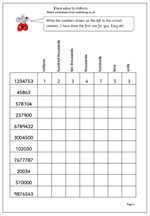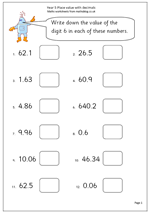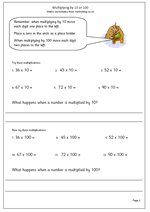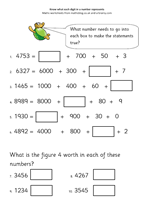## Place value to millionsMany children find reading large numbers very difficult, as indeed do many adults. This is a very simple page which looks at reading larger numbers up to millions. If nothing else it should help children realise that they need to start with hundreds tens and units and the the next three columns are then repeated but in thousands. A common error is to start trying to read a very large number from the largest digit when this can often just be a guess.

Working in blocks of three:

123 456 789

789 hundreds tens units

456 hundreds tens units of thousands

123 hundreds tens units of millions

so the number is 123 million 456 thousand and 789.

Easy!

Place value: millions

## Year 5 Maths worksheet: Place value with decimalsBy Year 5 children should have a good understanding of place value and know that the value of a digit is dependent on its place in the number. eg the 6 in 3.6 is six tenths, whilst the 6 in 16.4 is 6 units. This worksheet should demonstrate whether this concept has been understood. It asks that the value of the digit 6 is written down for each number shown, working with numbers up to to decimal places. This could be done as numbers or in words. Talk about the decimal fraction 0.6, that it can be read as six tenths or 6/10 and that 0.06 is six hundredths or 6/100.

Place value with decimals (pg 1)

## Year 4 maths worksheet: multiplying by 10 and 100Understanding what happens when you multiply a whole number by 10 is crucial to understanding maths at primary school and indeed with later maths. Children should have carried out lots of practical work with base 10 apparatus in school to show that:

when you multiply a whole number by 10 the digits move one place to the left.

Now this may give the appearance of ‘adding a nought’ as a zero is placed in the units. I know of many teachers and publishers who still use this expression, but it is extremely dangerous, especially when it comes to multiplying decimals by 10. (0.8 is not 0.80).

Multiplying by 100 is the same as multiplying by 10 and then multiplying by 10 again (10 x 10 = 100) so each digit will move two places to the left and zeros will need to be placed in the units and tens.

When you multiply a whole number by 100 the digits move two places to the left.

Multiplying by 10 and 100 (pg 1)

## Y4 free maths worksheet: place valueKnowing what each digit in a number represents can be a real challenge for children. Many do not understand that the digit 4 in 3400 has a different value to the digit 4 in 340. That is why understanding place value is so important once children begin to use larger numbers.

Here is a worksheet which should demonstrate whether place value has been understood. It asks what number needs to go into each box to make the number sentence correct. It shows that, for example:

2345 is made up of 2000 + 300 + 40 + 5

Place value (pg 1)